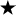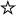#Chapter 2Simple Graphics## 2.6 Polygon

A Polygon is a collection of points used to create a series of line segments. Its primary purpose is to draw arbitrary shapes like triangles or pentagons. If the points are sufficiently close, you can create a curve. To display the Polygon, call drawPolygon() or fillPolygon().

### Polygon Methods

Variables

The collection of points maintained by Polygon are stored in three variables:

public int npoints

The npoints variable stores the number of points.

public int xpoints[]

The xpoints array holds the x component of each point.

public int ypoints[]

The ypoints array holds the y component of each point.

You might expect the Polygon class to use an array of points, rather than separate arrays of integers. More important, you might expect the instance variables to be private or protected, which would prevent them from being modified directly. Since the three instance variables are public, there is no guarantee that the array sizes are in sync with each other or with npoints. To avoid trouble, always use addPoints() to modify your polygons, and avoid modifying the instance variables directly. Constructors

public Polygon ()

This constructor creates an empty Polygon.

public Polygon (int xPoints[], int yPoints[], int numPoints)

This constructor creates a Polygon that consists of numPoints points. Those points are formed from the first numPoints elements of the xPoints and yPoints arrays. If the xPoints or yPoints arrays are larger than numPoints, the additional entries are ignored. If the xPoints or yPoints arrays do not contain at least numPoints elements, the constructor throws the run-time exception ArrayIndexOutOfBoundsException.

Miscellaneous methods

public void addPoint (int x, int y)

The addPoint() method adds the point (x, y) to the Polygon as its last point. If you alter the xpoints, ypoints, and npoints instance variables directly, addPoint() could add the new point at a place other than the end, or it could throw the run-time exception ArrayIndexOutOfBoundsException with a message showing the position at which it tried to add the point. Again, for safety, don't modify a Polygon's instance variables yourself; always use addPoint().

public Rectangle getBounds ()public Rectangle getBoundingBox ()The getBounds() method returns the Polygon's bounding Rectangle (i.e., the smallest rectangle that contains all the points within the polygon). Once you have the bounding box, it's easy to use methods like copyArea() to copy the Polygon.

getBoundingBox() is the Java 1.0 name for this method.

public boolean contains (int x, int y)public boolean inside (int x, int y)The contains() method checks to see if the (x, y) point is within an area that would be filled if the Polygon was drawn with Graphics.fillPolygon(). A point may be within the bounding rectangle of the polygon, but contains() can still return false if not within a closed part of the polygon.

inside() is the Java 1.0 name for this method.

public boolean contains (Point p)The contains() method checks to see if the point p is within an area that would be filled if the Polygon were drawn with Graphics.fillPolygon().

public void translate (int x, int y)The translate() method moves all the Polygon's points by the amount (x, y). This allows you to alter the location of the Polygon by shifting the points.